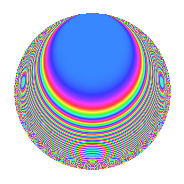# Properties

 Label 1340.2.aLevel 1340 Weight 2 Character orbit a Rep. character $$\chi_{1340}(1,\cdot)$$ Character field $$\Q$$ Dimension 22 Newforms 9 Sturm bound 408 Trace bound 7

# Learn more about

## Defining parameters

 Level: $$N$$ = $$1340 = 2^{2} \cdot 5 \cdot 67$$ Weight: $$k$$ = $$2$$ Character orbit: $$[\chi]$$ = 1340.a (trivial) Character field: $$\Q$$ Newforms: $$9$$ Sturm bound: $$408$$ Trace bound: $$7$$ Distinguishing $$T_p$$: $$3$$, $$7$$

## Dimensions

The following table gives the dimensions of various subspaces of $$M_{2}(\Gamma_0(1340))$$.

Total New Old
Modular forms 210 22 188
Cusp forms 199 22 177
Eisenstein series 11 0 11

The following table gives the dimensions of the cuspidal new subspaces with specified eigenvalues for the Atkin-Lehner operators and the Fricke involution.

$$2$$$$5$$$$67$$FrickeDim.
$$-$$$$+$$$$+$$$$-$$$$5$$
$$-$$$$+$$$$-$$$$+$$$$6$$
$$-$$$$-$$$$+$$$$+$$$$5$$
$$-$$$$-$$$$-$$$$-$$$$6$$
Plus space$$+$$$$11$$
Minus space$$-$$$$11$$

## Trace form

 $$22q$$ $$\mathstrut +\mathstrut 18q^{9}$$ $$\mathstrut +\mathstrut O(q^{10})$$ $$22q$$ $$\mathstrut +\mathstrut 18q^{9}$$ $$\mathstrut -\mathstrut 4q^{15}$$ $$\mathstrut +\mathstrut 6q^{17}$$ $$\mathstrut +\mathstrut 2q^{19}$$ $$\mathstrut -\mathstrut 6q^{23}$$ $$\mathstrut +\mathstrut 22q^{25}$$ $$\mathstrut -\mathstrut 24q^{27}$$ $$\mathstrut -\mathstrut 18q^{29}$$ $$\mathstrut -\mathstrut 8q^{33}$$ $$\mathstrut +\mathstrut 8q^{35}$$ $$\mathstrut +\mathstrut 10q^{37}$$ $$\mathstrut -\mathstrut 16q^{39}$$ $$\mathstrut -\mathstrut 12q^{41}$$ $$\mathstrut -\mathstrut 12q^{43}$$ $$\mathstrut -\mathstrut 8q^{45}$$ $$\mathstrut -\mathstrut 10q^{47}$$ $$\mathstrut +\mathstrut 42q^{49}$$ $$\mathstrut -\mathstrut 24q^{51}$$ $$\mathstrut +\mathstrut 20q^{53}$$ $$\mathstrut -\mathstrut 32q^{57}$$ $$\mathstrut -\mathstrut 26q^{59}$$ $$\mathstrut -\mathstrut 12q^{61}$$ $$\mathstrut +\mathstrut 8q^{65}$$ $$\mathstrut +\mathstrut 2q^{67}$$ $$\mathstrut +\mathstrut 8q^{69}$$ $$\mathstrut +\mathstrut 36q^{71}$$ $$\mathstrut -\mathstrut 6q^{73}$$ $$\mathstrut -\mathstrut 8q^{77}$$ $$\mathstrut -\mathstrut 24q^{79}$$ $$\mathstrut +\mathstrut 46q^{81}$$ $$\mathstrut -\mathstrut 24q^{83}$$ $$\mathstrut -\mathstrut 12q^{85}$$ $$\mathstrut +\mathstrut 12q^{87}$$ $$\mathstrut +\mathstrut 14q^{89}$$ $$\mathstrut +\mathstrut 16q^{91}$$ $$\mathstrut +\mathstrut 28q^{93}$$ $$\mathstrut -\mathstrut 8q^{95}$$ $$\mathstrut +\mathstrut 24q^{97}$$ $$\mathstrut -\mathstrut 12q^{99}$$ $$\mathstrut +\mathstrut O(q^{100})$$

## Decomposition of $$S_{2}^{\mathrm{new}}(\Gamma_0(1340))$$ into irreducible Hecke orbits

Label Dim. $$A$$ Field CM Traces A-L signs $q$-expansion
$$a_2$$ $$a_3$$ $$a_5$$ $$a_7$$ 2 5 67
1340.2.a.a $$1$$ $$10.700$$ $$\Q$$ None $$0$$ $$-2$$ $$-1$$ $$2$$ $$-$$ $$+$$ $$+$$ $$q-2q^{3}-q^{5}+2q^{7}+q^{9}+2q^{13}+\cdots$$
1340.2.a.b $$1$$ $$10.700$$ $$\Q$$ None $$0$$ $$1$$ $$-1$$ $$1$$ $$-$$ $$+$$ $$-$$ $$q+q^{3}-q^{5}+q^{7}-2q^{9}-6q^{11}+2q^{13}+\cdots$$
1340.2.a.c $$1$$ $$10.700$$ $$\Q$$ None $$0$$ $$1$$ $$-1$$ $$5$$ $$-$$ $$+$$ $$+$$ $$q+q^{3}-q^{5}+5q^{7}-2q^{9}+2q^{13}+\cdots$$
1340.2.a.d $$2$$ $$10.700$$ $$\Q(\sqrt{17})$$ None $$0$$ $$-1$$ $$2$$ $$-3$$ $$-$$ $$-$$ $$+$$ $$q-\beta q^{3}+q^{5}+(-2+\beta )q^{7}+(1+\beta )q^{9}+\cdots$$
1340.2.a.e $$2$$ $$10.700$$ $$\Q(\sqrt{3})$$ None $$0$$ $$2$$ $$2$$ $$4$$ $$-$$ $$-$$ $$-$$ $$q+(1+\beta )q^{3}+q^{5}+2q^{7}+(1+2\beta )q^{9}+\cdots$$
1340.2.a.f $$3$$ $$10.700$$ $$\Q(\zeta_{14})^+$$ None $$0$$ $$-2$$ $$3$$ $$-2$$ $$-$$ $$-$$ $$+$$ $$q+(-1-\beta _{2})q^{3}+q^{5}+(1-3\beta _{1}+2\beta _{2})q^{7}+\cdots$$
1340.2.a.g $$3$$ $$10.700$$ 3.3.257.1 None $$0$$ $$4$$ $$-3$$ $$-2$$ $$-$$ $$+$$ $$+$$ $$q+(1+\beta _{1})q^{3}-q^{5}+(-1+\beta _{1}+\beta _{2})q^{7}+\cdots$$
1340.2.a.h $$4$$ $$10.700$$ 4.4.60513.1 None $$0$$ $$-1$$ $$4$$ $$5$$ $$-$$ $$-$$ $$-$$ $$q-\beta _{1}q^{3}+q^{5}+(1+\beta _{3})q^{7}+(1+\beta _{1}+\cdots)q^{9}+\cdots$$
1340.2.a.i $$5$$ $$10.700$$ 5.5.1465016.1 None $$0$$ $$-2$$ $$-5$$ $$-10$$ $$-$$ $$+$$ $$-$$ $$q-\beta _{1}q^{3}-q^{5}+(-2+\beta _{2})q^{7}+(2+\beta _{1}+\cdots)q^{9}+\cdots$$

## Decomposition of $$S_{2}^{\mathrm{old}}(\Gamma_0(1340))$$ into lower level spaces

$$S_{2}^{\mathrm{old}}(\Gamma_0(1340)) \cong$$ $$S_{2}^{\mathrm{new}}(\Gamma_0(20))$$$$^{\oplus 2}$$$$\oplus$$$$S_{2}^{\mathrm{new}}(\Gamma_0(67))$$$$^{\oplus 6}$$$$\oplus$$$$S_{2}^{\mathrm{new}}(\Gamma_0(134))$$$$^{\oplus 4}$$$$\oplus$$$$S_{2}^{\mathrm{new}}(\Gamma_0(268))$$$$^{\oplus 2}$$$$\oplus$$$$S_{2}^{\mathrm{new}}(\Gamma_0(335))$$$$^{\oplus 3}$$$$\oplus$$$$S_{2}^{\mathrm{new}}(\Gamma_0(670))$$$$^{\oplus 2}$$